SOLSTICE: An Electronic Journal of Geography and Mathematics. (Major articles are refereed; full electronic archives available). Persistent URL:  http://deepblue.lib.umich.edu/handle/2027.42/58219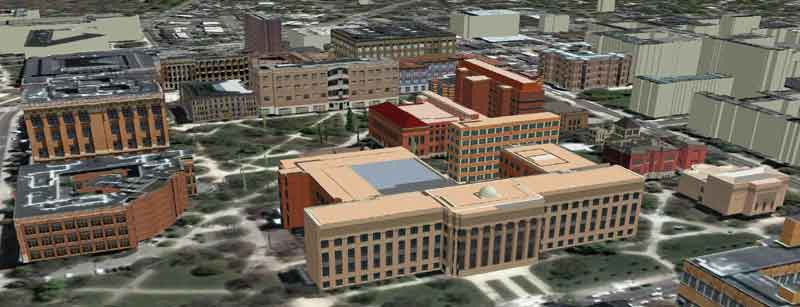Best viewed in Firefox browser (but should work in any current browser).  Works best with a high speed internet connection.
Final version of IMaGe logo created by Allen K. Philbrick from original artwork from the Founder.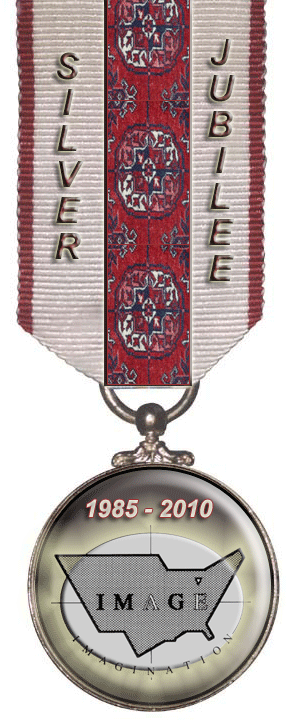Fractals Take A Non-Euclidean
Central Place
Sandra L. Arlinghaus, Ph.D., The University of Michigan

Introduction

Central place geometry looks at groupings of hexagons as they tile the Euclidean plane.  Pattern within these groupings may be interpreted as representing various elements of how cities share space.  The geometry plays out over the unbounded surface of the Euclidean plane; the interpretation surface, however, involves the bounded surface of the Earth (roughly a sphere).  One might ask if tilings of regular polygons could suggest other surfaces as a basis for similar interpretation.
Euclid's Fifth ("Parallel") Postulate states that given a line, and a point not on that line, there is exactly one line through that point that does not intersect the given line.  That is, the newly drawn line is "parallel" to the given line.
In "Non-Euclidean" geometries the Parallel postulate does not hold (Coxeter, 1961, 1965).  One of these non-Euclidean geometries, that admits multiple rather than unique parallels, is called "hyperbolic" geometry.  For inspiration for that name, consider  the hyperbola:  multiple branches through a single point not on a given line might all be asymptotic to the given line--they do not intersect it.  Of course, as the process becomes infinite, one might imagine the asymptote getting to the line--of the "parallels" meeting at a point at infinity.
Most of us live in an Euclidean universe.  We may function in other ways, but when we attempt to capture the geometry of our lives we do so, for the most part, in the Euclidean plane.  To do otherwise, is jarring to most.

Conventional Central Place Geometry:  Fractal Characterization

The patterns that emerge from grouping sets of hexagons according to K=3, K=4, and K=7 principles are familiar to even beginning geography students.  What is perhaps not as familiar is the use of fractal geometry to produce these patterns from number theoretic properties (Arlinghaus, 1985; Arlinghaus and Arlinghaus, 1989).   See the linked materials for a full explanation; Figures 1.a, 1.b, and 1.c show (respectively) how to create a generator to form appropriate pattern for the K=7, K=4, and K=3 hierarchies.  Iteration of the generator at different geographic scales produces the entire landscape as suggested in Figure 1.d which shows the next level of generator scaling and iteration (Arlinghaus and Arlinghaus, 2005).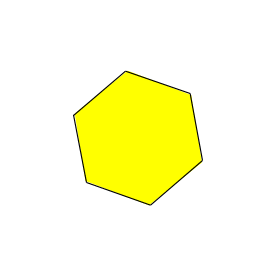Figure 1.a.  K=7 central place hierarchy:  fractal creation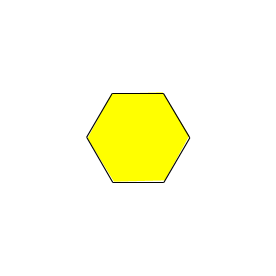Figure 1.b.  K=4 central place hierarchy:  fractal creation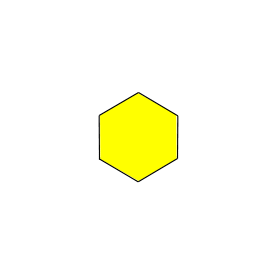Figure 1.c.  K=3 central place hierarchy:  fractal creation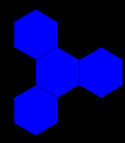Figure 1.d.  Demonstration of iteration of fractal generator for K=4 hierarchy.

An advantage to the fractal approach is that it permits complete systematic extension of process to higher K-values and, at the same time, allows complete solution to existing open questions.  One broad concern, however, that is not addressed in this approach or in any other within the Euclidean plane is the issue of bounding the process (rather than allowing it to continue to indefinite extent).  In the world of fine art, Maurits Escher addressed this issue elegantly with his Circle Limit Series in which pattern that replicates indefinitely is confined within the boundary of a "limiting" circle.

The Hyperbolic Plane:  Poincaré Disk Model

In fact, what Escher did was to embed his interesting carved polygons in the Poincaré Disk model of the non-Euclidean geometry called hyperbolic geometry.  The disk contains the entire hyperbolic plane--the tiles appear, from our Euclidean vantage point in the plane of the paper, to disappear as one moves farther toward the limit of the circle edge.  Sides of the tiles appear curved, again from our Euclidean standpoint.   It is easy to understand this idea (on the sphere) simply by placing a polygon on the Google Earth globe and sliding it around---the animation in Figure 2 illustrates this idea.  The reader may download the associated .kmz file here to try it for himself.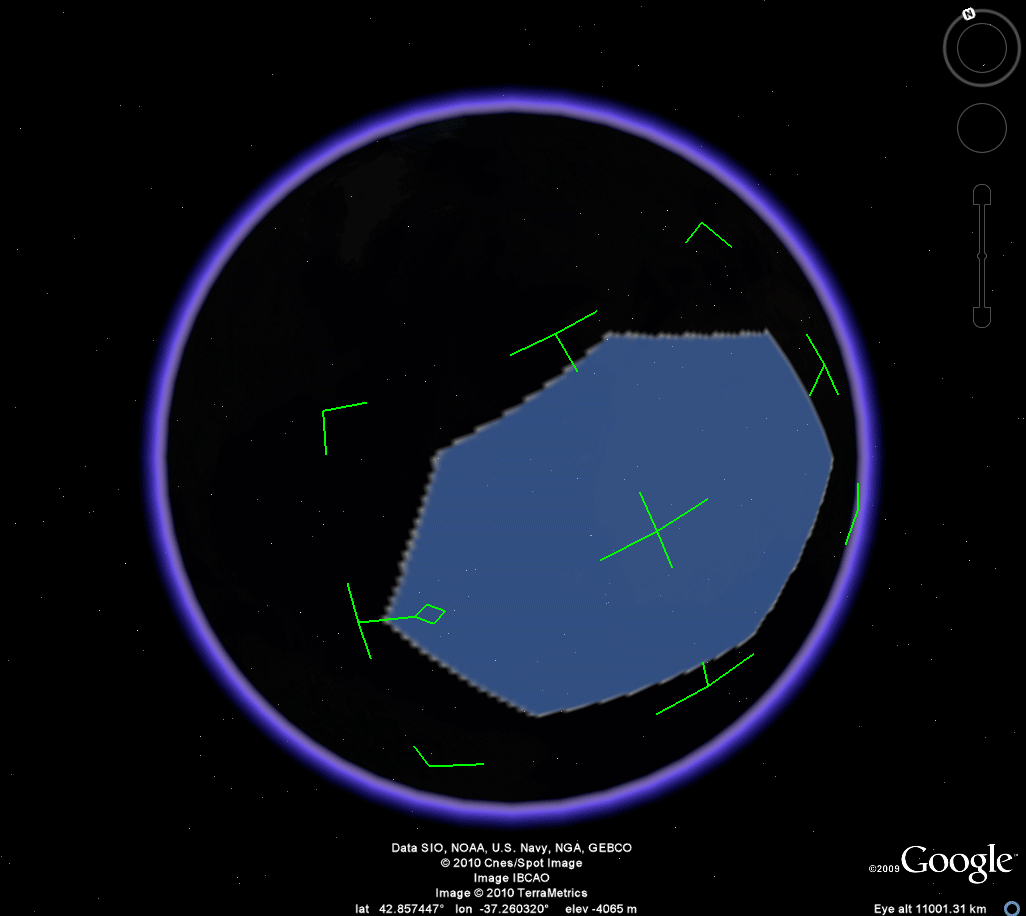Figure 2.

The difference between the polygon on the sphere and a polygon in the hyperbolic plane is that the sphere has constant positive curvature whereas the hyperbolic plane has constant negative curvature, so one must imagine sides that are convex (bulge out) on the sphere would be sides that are concave (sink inward) in the hyperbolic plane (and vice-versa).  With the curvature issue, and the shrinking of polygons with distance from the center, in mind, a natural question is to ask about the nature of tilings of this new space.

The Hyperbolic Plane:  Tilings

Data compression and the Fibonacci sequence

The Fibonacci sequence is a number pattern that has seen, and continues to see, application in a number of different arenas.  The sequence itself is generated as follows:
Start with 1; add the preceding number to it (there is none) generating the next term which is also 1.  Then add this new 1 to the previous term generating 2; then add 2 and 1, and so forth, to create the following number pattern:

1, 1, 2, 3, 5, 8, 13, 21, 34, 55, 89, 144,...

Some characterizations of this sequence simply have it begin as 1, 2, 3, 5, 8, 13, 21, 34, 55, 89, 144,...  Whichever is used, its applications are deep and well known.  Indeed, an entire journal, The Fibonacci Quarterly, is devoted to its theory and applications.  Using it to represent phyllotaxis and the arrangement of spiral leaf patterns on branches or the spiral arrangement of cells on the skin of a pineapple have been with us for a long time.  So too has an interpretation of the sequence in terms of the way that rabbits reproduce.

More recent applications employ the sequence in creating a universal computer code that will stem error propogation in computing systems.  This property, and others, classify Fibonacci coding as a "Lossless" Entropy Encoding Data Compression Method.

Pattern compression in the hyperbolic plane and the Fibonacci sequence

Series and Wright note that tilings, as well, can create interesting methods of data compression through pattern compression.  They note the following example involving similar tilings of both the Euclidean plane, Figure 3.a, and the Hyperbolic plane (realized in the Poincaré Disk Model), Figure 3.b.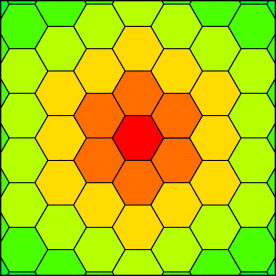Figure 3.a.  Tiling of the Euclidean plane by hexagons.  Figure appeared originally in Series and Wright, http://plus.maths.org/issue43/features/serieswright/index.html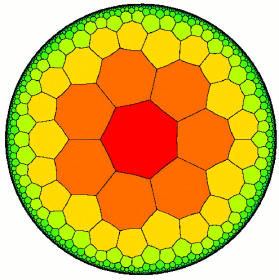Figure 3.b.  Tiling of the hyperbolic plane, realized as the Poincaré Disk Model, by heptagons (7-sided polygons).  Figure appeared originally in Series and Wright, http://plus.maths.org/issue43/features/serieswright/index.html

Simple enumeration of bands of polygons surrounding a chosen central polygon reveals a numerical compression of pattern.  Of particular interest, note that once again the Fibonacci sequence gives a systematic basis for the greater numerical compression available in the hyperbolic plane (Series and Wright).  Figure 4.a shows the simple linear number pattern that emerges from the hexagonal tiling of the Euclidean plane while Figure 4.b captures systematically the pattern in the hyperbolic plane.

 n Tiles in nth layer 1 6 2 12 3 18 4 24 5 30 6 36 7 42 8 48 9 54 10 60 11 66 12 72

Figure 4.a.  Tiling of the Euclidean plane by hexagons.  Sequence of tiles in nth layer is the number in a polygonal cell (six) times n.  Thus, for example, when n=4, there are 6*4=24 tiles in the fourth layer out from the center.
 n Tiles in nth layer 2nth Fibonacci Number 1 7 1 2 21 3 3 56 8 4 147 21 5 385 55 6 1008 144 7 2639 377 8 6909 987 9 18088 2584 10 47355 6765 11 123977 17711 12 324576 46368

Figure 4.b.  Tiling of the hyperbolic plane, realized as the Poincaré Disk Model, by heptagons (7-sided polygons).  Sequence of tiles in the nth layer is the number of sides in a polygonal cell (seven) times the 2nth Fibonacci number.  Thus, for example, when n=4, the 2nth Fibonacci number is 21 so that there are 7*21 = 147 tiles in the 4th layer out from the center.

Pattern compression in the hyperbolic plane is more than 4500 fold greater than in the Euclidean plane at the level of n=12 and this compression rate appears to increase as n increases (Figure 5).

 n Compression fold 1 1.166666667 2 1.75 3 3.111111111 4 6.125 5 12.83333333 6 28 7 62.83333333 8 143.9375 9 334.962963 10 789.25 11 1878.439394 12 4508

Figure 5.  Demonstration of the increase in compression as n increases.

It remains to offer formal proof of this observation; however, it seems clear that the observation is true since the Fibonacci sequence itself, let alone the 2nth Fibonacci sequence, becomes large faster than does the sequence of natural numbers.
Central Place Hierarchies in the Hyperbolic Plane

Possible tilings
While the observation by Series and Wright concerning the Fibonacci sequence in association with an heptagonal tiling of the hyperbolic plane appears both interesting and important, one might ask about other tilings of the plane and such association with the Fibonacci sequence, or other ideas, as a compression tool.  An article in Wikipedia is useful in classifying possible tilings of the hyperbolic plane as realized in the Poincaré Disk Model.  The reader is encourage to take a look at the link above and study the beautiful and highly symmetric patterns that emerge.

Central Place Theory
One of the "other ideas" that seems natural to consider as a compression tool is that of the fractal.  Thus, a logical next step might be to try to merge the fractal compression from central place theory (and hexagonal tilings of the Euclidean plane) with a corresponding construction in the hyperbolic plane (Poincaré Disk Model) in order to assign fractal dimension to these patterns as ways to analyze the extent to which space is "filled" by them in the limiting process.

Tiling by heptagons
In the spirit of the example from Series and Wright, begin by considering the heptagonal tiling of the hyperbolic plane.  Figure 6.a shows selection of a generator, similar to the generator for K=7 in the Euclidean case above (Figure 1.a) that will generate, when scaled appropriately and applied throughout the Disk, the entire network mesh.  Here, the generator has four sides; in the corresponding Euclidean case the generator had 3 sides.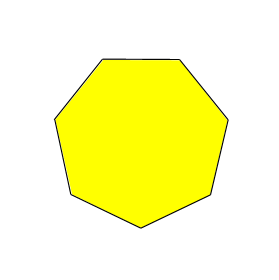Figure 6.a.  Fractal generation of pattern shown in Figure 6.b.  The generator has four sides.Figure 6.b.  Figure 3.b is repeated here for ease in visual reference with Figure 6.a.
The simplest hierarchy to consider is the one in which the natural ring of surrounding polygons needs to be produced:  in the Euclidean case with hexagonal tiling of the plane, that is K=7 in which a central hexagon is surrounded by 6 others (for 7=6+1).  In the hyperbolic case with heptagonal tiling of the plane, a natural extension is to refer to the pattern as K=8 (for 8=7+1).  One of the reasons to capture central place pattern as a fractal involved a desire to be able to assign a single number, a fractal (Hausdorff-Besicovitch) dimension D, to characterize the entire hierarchy.  For the Euclidean case, the formula based on work of Mandelbrot, used log(number of generator sides) / log (square root of K).  Thus, when K=7 in the Euclidean case, the generator with 3 sides yielded a value for D of 1.1291501...  For the generator of 4 sides (Figure 6.a) in the K=8 hierarchy, the value of D = LOG10(4)/LOG10(SQRT(8)) = 1.333333..., if one extends this formula to the hyperbolic plane.   Under this latter assumption, clearly more space is filled in the hyperbolic case than it is in the associated Euclidean space:  again, compression is greater in the hyperbolic than in the Euclidean world.

To continue the central place theory extension, one might ask about the cases for K=4 and K=3.  A natural appoach, for K=4 for example, is to consider using as a generator one that surrounds half the area of a heptagon in the hyperbolic plane.  Then, apply this generator in an "in and out" fashion to eventually generate a set of polygons that will cover 7*0.5 + 1 amount of heptagonal cells.  The problem with this approach is that the "in and out" approach requires an even number of sides in the basic cell; otherwise, two "outs" are adjacent and the inner central cell becomes the wrong shape.  Thus, the hierarchy cannot be produced.  Figure 7 shows the beginning of this application of a natural generator, with reference to the underlying base so that the difficulty with iteration is clearer.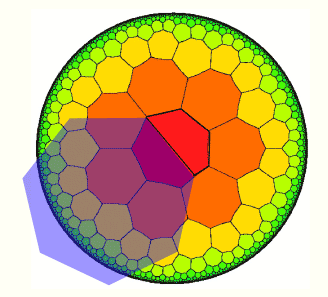Figure 7.  Lack of iterative capability of naturally selected fractal generator.

Prime numbers and polygon shape
Clearly one cannot examine each individual case, as with Figures 6 and 7, to determine when a particular tiling might or might not be generated through fractal generation.  One easy approach to this problem is to look at the number-theoretic properties of the base cell of the tiling.  In the case of hexagons, the unique factorization of 6 is 3*2.  Thus, one can look to generating 1/3 (K=3) and 1/2 (K=4) polygonal pieces in association with fractal iteration.  In the case of a prime number, such as 7, one can find only the natural surrounding pattern of full tiles--no fractional tiles.

With this idea in mind, move now to considering other tilings of the hyperbolic plane as candidates for calculation of fractal dimension.  A natural place to begin is with tiling by hexagons.

Tiling by hexagons
One of the characteristics that a central place style of tiling has is that cells on each side of a central cell touch other cells with a side in common with that central cell.  Thus, the tiling by hexagons shown in Figure 8 is not this sort of tiling:  tiles 1 and 2 are in correct position in relation to the central cell and to each other.  So also are the polygon pairs 3 and 4, as well as 5 and 6.  However 2 and 3 are separated by an x cell, as are 4 and 5, as well as 6 and 1.  The required adjacency pattern is only partially present and so this case is discarded (for now) as not a true related central place case.  Thus, one sees why Series and Wright chose the heptagonal tiling, rather than an hexagonal tiling, of the hyperbolic plane as the one naturally associated with the hexagonal tiling of the Euclidean plane.  One could, of course, choose other tilings (Woldenberg, 1968).  The point here is to indicate what tiling of the hyperbolic plane is, or is not, similar to a tiling of the Euclidean plane by hexagons.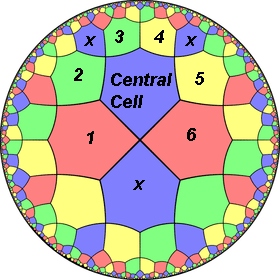Figure 8.  Hexagonal tiling not of central place net type.  http://en.wikipedia.org/wiki/Uniform_tilings_in_hyperbolic_plane http://creativecommons.org/licenses/by-sa/3.0/

Tiling by pentagons
As in the case above, there are gaps separating tiles.  This time, not in pairs but between every tile surrounding the central tile.  Figure 9 illustrates this difficulty (which is more extreme than in Figure 8) using notation parallel to that employed in Figure 8.  Again, as with the hexagonal tiling, this case which might have appeared attractive at the outset, is discarded as well:  its level of violation of a basic premise is even more extreme than is the case with the hexagonal tiling.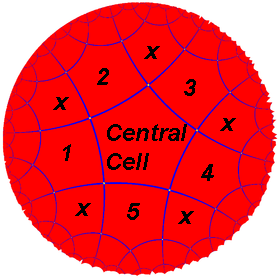Figure 9.  Pentagonal tiling not of central place net type. http://en.wikipedia.org/wiki/Uniform_tilings_in_hyperbolic_plane http://creativecommons.org/licenses/by-sa/3.0/

Tiling by octagons
The tiling of the hyperbolic plane shown in Figure 10 is not even as close to a central place net as those in either Figure 8 or Figure 9.  Here, the central octagonal cell is surrounding by 8 cells with correct adjacency but they are hexagonal rather than octagonal cells.  The next layer out has octagonal cells with no adjacency--each pair of octagonal cells is separated by an hexagonal cell.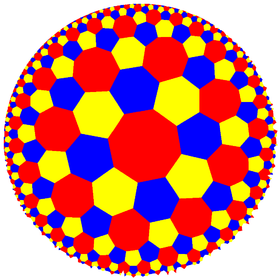Figure 10.  Octagonal tiling not of central place net type. http://en.wikipedia.org/wiki/Uniform_tilings_in_hyperbolic_plane http://creativecommons.org/licenses/by-sa/3.0/

It remains an open question to determine if there are any other true central place net styles of tiling of the hyperbolic plane (there were with the Euclidean plane however there only the case of square tiles (Arlinghaus, 1992) is of interest since a hexagon may be decomposed into triangles).  Examination of cases did suffice in the Euclidean case and it may well be sufficient in the hyperbolic case, as well--a quick examination of the visual pattern displayed in the Wikipedia article suggests no others; however, a more systematic search, based on careful notational analysis rather than solely on visual pattern, is necessary to prove there are no others.  Indeed, that is one direction for the future--to prove uniqueness of a single central place net for hyperbolic space.

For the Future...
• Uniqueness paves the way for canonical form
• Generating function
• Extensions to different mixed and other tilings (as, conceptually, in earlier work of Woldenberg, 1968; Arlinghaus, 1991)
• Extensions to higher order places
• Graph theory and duality
• Interpretations, focusing perhaps on pattern compression for urban areas, networks, and elsewhere.
Related Materials

General links (last accessed June 15, 2010) and references:

• Arlinghaus, Sandra L.  1991.  Essays on Mathematical Geography:  III.  Ann Arbor:  Institute of Mathematical Geography.  Link
• Arlinghaus, Sandra L. 1985.  "Fractals take a central place," Geografiska Annaler, 67B, pp. 83-88. Journal of the Stockholm School of Economics.
• Arlinguaus, Sandra L. and Arlinghaus, William C.  2005.  Spatial Synthesis:  Volume I, Centrality and Hierarchy, Book 1.  Ann Arbor:  Institute of Mathematical Geography.  Link
• Arlinghaus, Sandra L. and Arlinghaus, William C.  1989.  "The fractal theory of central place hierarchies: a Diophantine analysis of fractal generators for arbitrary Löschian numbers," Geographical Analysis: an International Journal of Theoretical Geography. Ohio State University Press. Vol. 21, No. 2, April, 1989; pp. 103-121.
• Coxeter, H. S. M.  1961, 1965 (fourth printing).  Introduction to Geometry.  New York:  John Wiley and Sons.
• Coxeter, H. S. M.  1961 (fourth edition). Non-Euclidean Geometry.  Toronto:  University of Toronto Press.
• Escher, M. C.  Circle Limit Series.  A variety of references may be found using a current search engine:  one such is found at this link.
• Peterson, Ivars.  2001.  Visions of Infinity.  Science News Online  Found at:  http://www.sciencenews.org/articles/20001223/bob8.asp
• Series, Caroline and Wright, David.  Non-Euclidean Geometry and Indra's Pearls.  http://plus.maths.org/issue43/features/serieswright/index.html
• Wikipedia, Fibonacci coding,  http://en.wikipedia.org/wiki/Fibonacci_coding
• Wikipedia, Uniform tilings in hyperbolic plane, http://en.wikipedia.org/wiki/Uniform_tilings_in_hyperbolic_plane
• Woldenberg, Michael.  1968.  Hierarchical Systems, Cambridge, Mass : Laboratory for Computer Graphics and Spatial Analysis, Harvard Center for Environment Desing Studies, The Graduate School of Desing Harvard University.  Link
 .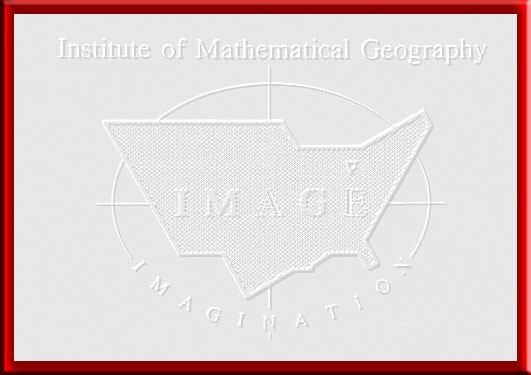Solstice:  An Electronic Journal of Geography and Mathematics,  Volume XXI, Number 1 Institute of Mathematical Geography (IMaGe). All rights reserved worldwide, by IMaGe and by the authors. Please contact an appropriate party concerning citation of this article: sarhaus@umich.edu http://www.imagenet.org http://deepblue.lib.umich.edu/handle/2027.42/58219 Solstice was a Pirelli INTERNETional Award Semi-Finalist, 2001 (top 80 out of over 1000 entries worldwide) One article in Solstice was a Pirelli INTERNETional Award Semi-Finalist, 2003 (Spatial Synthesis Sampler). Solstice is listed in the Directory of Open Access Journals maintained by the University of Lund where it is maintained as a "searchable" journal. Solstice is listed on the journals section of the website of the American Mathematical Society, http://www.ams.org/ Solstice is listed in Geoscience e-Journals IMaGe is listed on the website of the Numerical Cartography Lab of The Ohio State University:  http://ncl.sbs.ohio-state.edu/4_homes.html Congratulations to all Solstice contributors. Remembering those who are gone now but who contributed in various ways to Solstice or to IMaGe projects, directly or indirectly, during the first 25 years of IMaGe: Allen K. Philbrick | Donald F. Lach | Frank Harary | H. S. M. Coxeter | Saunders Mac Lane | Chauncy D. Harris | Norton S. Ginsburg | Sylvia L. Thrupp | Arthur L. Loeb | George Kish |